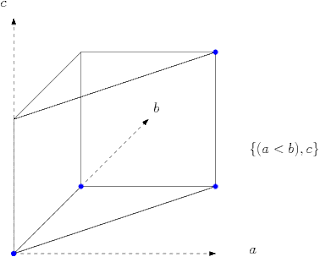## Monday, March 12, 2007

### Order polytopes

One view of the techniques of computational (and combinatorial) geometry is the delicate tension between the discrete (combinatorial) and the continuous (geometric). Whether it be Davenport-Schinzel sequences and lower envelopes, or VC-dimension and intersections of geometric sets, a common step in making geometric structures tractable is finding a combinatorial structure that captures the aspect of the geometry that's important for a particular problem.

It's nice to return the favor sometimes. Combinatorics and topology have strong connections, and one doesn't have to go too far from home find instances of "lifting" a combinatorial problem to a topological domain to solve it; Lovasz's proof of the Kneser conjecture, and the various methods for proving evasiveness properties, are among the more well known examples. In fact, distributed computing is replete with topological hammers for proving properties of protocols.

Of course, one can view the entirety of linear programming as an example of lifting combinatorics to geometry; I'm deliberately ignoring it because you have to go through the integer lattice to get combinatorial problems, rather than having geometry characterizing the structure directly. This is of course totally ad hoc :)

What I describe next is an extremely elegant geometric view of a purely combinatorial problem. Suppose you're sorting numbers, and you have some rough idea of the sorted order. Maybe you can rank some of the elements, but not all of them (for example, maybe you're integrating results from different web searches). At any rate, what you have is a partial order, and you'd like to get a sense of its 'orderedness'. Specifically, you'd like to estimate the number of different ways this partial order can be completed (by inserting orderings between as-yet unordered elements without disturbing existing order). Such a completion (or extension) is called a linear extension, because you can think of the final total order as being points on a line, numbered from lowest to highest rank.

For example, suppose you have the four elements (a,b,c,d) and you know that (a < b) and (c < d). There are then six ways of constructing a linear extension from this partial order.

Suppose now that comparisons were costly, and we'd like to choose the next comparison so as to reduce the number of potential linear extensions by as much as possible. If we could guarantee that the number reduced by an α fraction, then we could complete the sorting using logα E comparisons, where E was the number of linear extensions to begin with. Since each comparison partitions the set of linear extensions into two sets, clearly this fraction must be at least 1-α.

It's not hard to see that we can't do better than α = 2/3, by considering the partial order {(a < b), c}. There's always a way of picking the answer to the next comparison to make this so. The best known bound to date is roughly α = 0.7236, and this bound (and earlier methods) make use of an elegant geometric characterization of linear extensions via order polytopes.

Given n elements in the partial order, let the order polytope be the set of points in [0,1]n such that the coordinates of each point satisfies the partial order. Namely, if there's a constraint of the form a1 < a2, then the coordinates x1 and x2 of the point x satisfy the same constraint.

It's not hard to see that this is indeed a polytope; it's the intersection of a set of hyperplanes, and is bounded. What is more intriguing is that every simplex of this polytope corresponds to a specific linear extension.If we look for example at the simplex defined by the four blue points, the coordinates are (0,0,0), (0,1,1), (0,1,0), and (1,1,1), which describes the order (a < c < b). All these simplices are congruent and non overlapping, and each has volume 1/n!. This implies the remarkable result that the number of linear extensions for a given partial order is the volume of the resulting order polytope, divided by n!.

The favor gets returned as well. The paper, "Counting linear extensions is #P-complete", by Brightwell and Winkler in 1991, yields the corollary that computing the volume of a polyhedron is #P-complete.

For more on this, Matousek's Lectures on Discrete Geometry is a wonderfully readable source.

#### 1 comment:

1.Awesome results. Thanks for the exposition.